Courses

# Past Year Questions: Deflection of Beams Notes | EduRev

## Civil Engineering (CE) : Past Year Questions: Deflection of Beams Notes | EduRev

The document Past Year Questions: Deflection of Beams Notes | EduRev is a part of the Civil Engineering (CE) Course Topic wise GATE Past Year Papers for Civil Engineering.
All you need of Civil Engineering (CE) at this link: Civil Engineering (CE)

Q.1 The figure shows a simply supported beam PQ of uniform flexural rigidity El carrying two moments M and 2M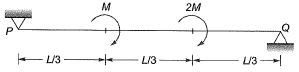The slope at P will be    [2018 : 2 Marks, Set-I]
(a) 0
(b) ML/(9EI)
(c) ML/(6EI)
(d) ML/(3EI)
Ans.
(C)
Solution:
Method-l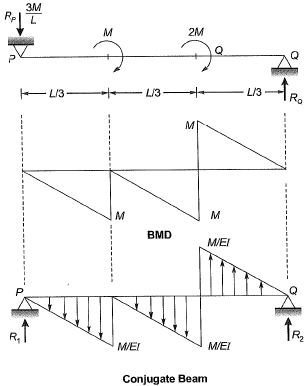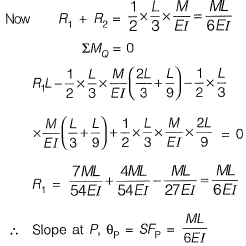Method-ll
Moment area method: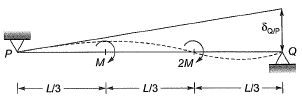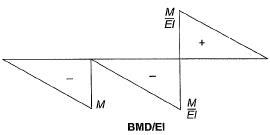δQ/P = Defleciton of point Q wrt to tangent at point P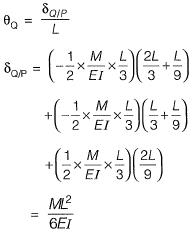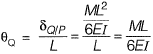Q.2 Two prismatic beams having the same flexural rigidity of 1000 kN-m2 are shown in the figures.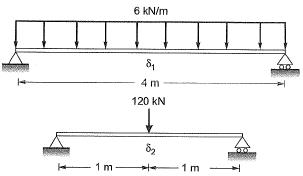If the mid-span deflections of these beams are denoted by δ1 and δ2 (as indicated in the figures), the correct option is    [2017 : 2 Marks, Set-II]
(a) δ1 = δ2
(b) δ1 < δ2
(c) δ> δ2
(d) δ1 >> δ2
Ans.
(A)
Solution: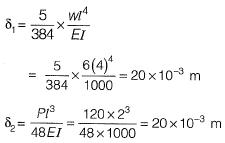∴ δ= δ2

Q.3 Two beams PQ (fixed at P with a roller support at Q, as shown in Figure I, which allows vertical movement) and XZ(with a hinge at Y) are shown in the Figures I and II respectively. The spans of PQ and XZ are L and 2L respectively. Both the beams are under the action of uniformly distributed load (w) and have the same flexural stiffness, EI (where, E and I respectively denote modulus of elasticity and moment of inertia about axis of bending). Let the maximum deflection and maximum rotation be δmax1 and δmax1, respectively, in the case of beam PQ and the corresponding quantities for the beam XZ be δmax2 and δmax2, respectively.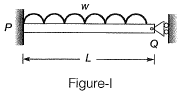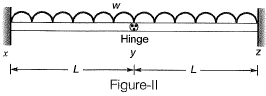Which one of the following relationship is true?    [2016 : 2 Marks, Set-I]
(a)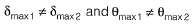(b)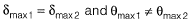(c)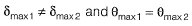(d)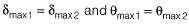Ans. (D)
Solution: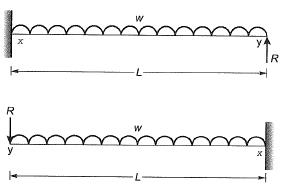Deflection in beam xy at y = Deflection in beam yz at y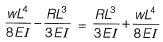∴ R = 0
In beam PQ also at support Q, vertical reaction in zero because of roller support.
So, beam PQ, xyand yz are same.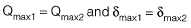Q.4 A 3 m long simply supported beam of uniform cross -section is subjected to a uniformly distributed load of w= 20 kN/m in the central 1 m as shown in the figureIf the flexural rigidity (El) of the beam is 30 x 106 N-m2, the maximum slope (expressed in radians) of the deformed beam is    [2016 : 2 Marks, Set-I]
(a) 0.681 x 10-7
(b) 0.361 x 10-3
(c) 4.310 x 10-7
(d) 5.910 x 10-7
Ans.
(B)
Solution:
Method-1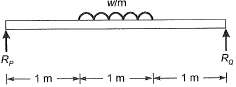Due to symmetrical loading,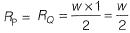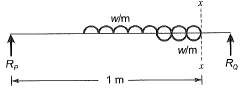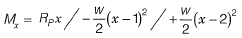According to Macaulay method,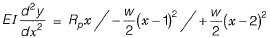After integrating once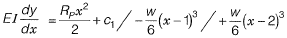Due to symmetrical loading slope will be zero at mid section (x = 1.5 m),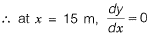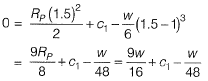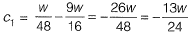∴ Equation of slope,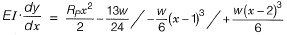The slope will be maximum at the support,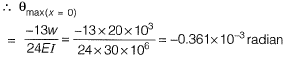Method-ll
Moment Area Method,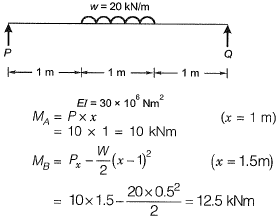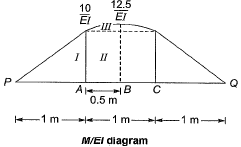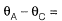Area of M/El diagram between points P and B.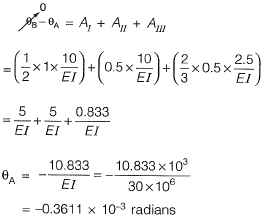Q.5 Two beams are connected by a linear spring as shown in the following figure. For a load P as shown in the figure, the percentage of the applied load P carried by the spring is_____.    [2015 : 2 Marks, Set-I]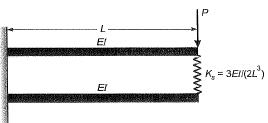Solution: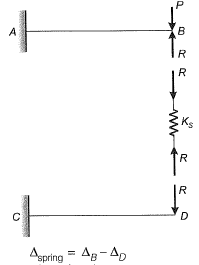Compression of spring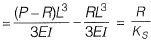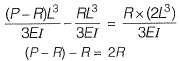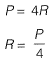% force carried by spring = 25%

Q.6 A steel strip of length, L = 200 mm is fixed at end A and rests at 6 on a vertical spring of stiffness, k = 2 N/mm. The steel strip is 5 mm wide and 10 mm thick. A vertical load, P= 50 N is applied at 6, as shown in the figure. Considering E = 200 GPa, the force (in N) developed in the spring is ________ .    [2015 : 2 Marks, Set-II]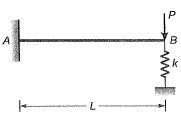Solution: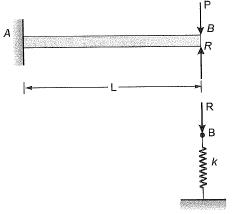Deflection of point B = Deflection of spring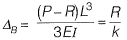Where, R= Force in the spring,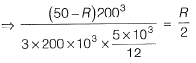0.064(50 - R) = R
3.2= R+ 0.064 R
R = 3.0075 N

Q.7 A horizontal beam ABC is loaded as shown in the figure below. The distance of the point of contraflexure from end A (in m) is _______ .    [2015 : 1 Mark, Set-II]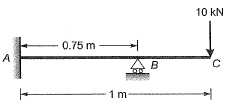Solution:

Reaction at B,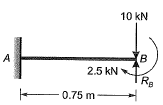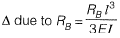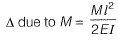ΔB = 0 (Compatibility condition)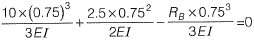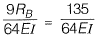∴ RB = 15 kN
BM at a distance x from free end,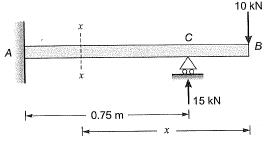BMx = 10 * x - 15 x (x - 0,25)= 0
⇒ 10x = 15x-3.75
⇒ 5x = 3.75
∴ x = 0.75m
∴ From end A, distance is 0.25 m.

Q.8 The beam of an overall depth 250 mm (shown below) is used in a building subjected to two different thermal environments. The temperatures at the top and bottom surfaces of the beam are 360C and 720C respectively. Considering coefficient of thermal expansion (α) as 1.50 x 10-5 per 0C, the vertical deflection of the beam (in mm) at its midspan due to temperature gradient is _______ .    [2014 : 2 Marks, Set-II]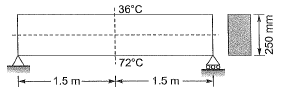Solution:
Method-I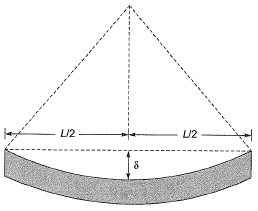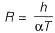From properties of circle,(Considering ‘δ’ very small so neglect δ2)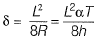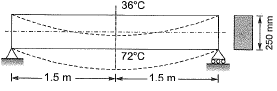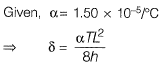= 2.43
Method-ll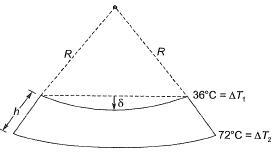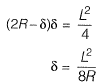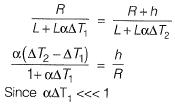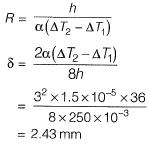Q.9 The tension (in kN) in a 10m long cable, shown in the figure, neglecting its self-weight is    [2014 : 2 Marks, Set-II]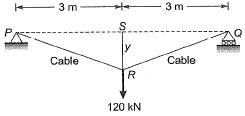(a) 120
(b) 75
(c) 60
(d) 45
Ans. (B)
Solution: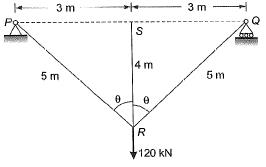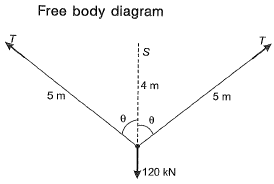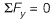⇒ 2T cosθ = 120 ...(i)
Here,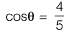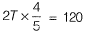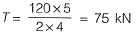Q.10 The axial load (in kN) in the member PQ for the arrangement/assembly shown in the figure given below is _________.    [2014 : 2 Marks, Set-II]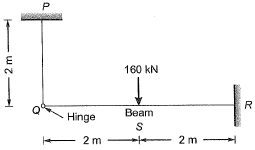Solution:

Free body diagram,
For principle of superposition,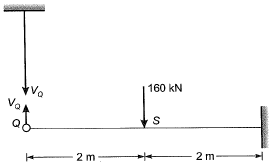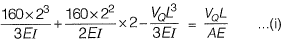Deflections due to axial forces will be very less as compared to bending forces.
So we can neglect the axial deformation.
∴ From equation (i),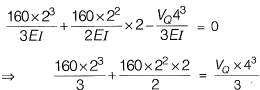⇒ VQ = 50 kN

Q.11 For the cantilever beam of span 3 m (shown below), a concentrated load of 20 kN applied at the free end causes a vertical displacement of 2 mm at a section located at a distance of 1 m from the fixed end. If a concentrated vertically downward load of 10 kN is applied at the section located at a distance of 1 m from the fixed end (with no other load on the beam), the maximum vertical displacement in the same beam (in mm) is ____.    [2014 : 2 Marks, Set-I]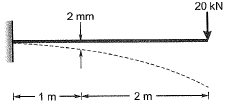Solution: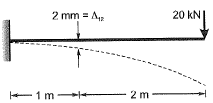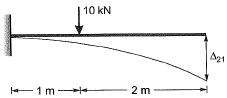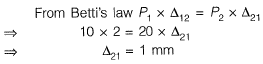Q.12 A uniform beam (EI= constant) PQ in the form of a quarter circle of radius R is fixed at end P and free at the end Q, where a load H/is applied as shown. The vertical downward displacement δQ at the loaded point Q is given by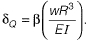Find the value of β correct to 4-decimal place.    [2013 : 2 Marks]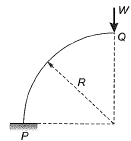Solution: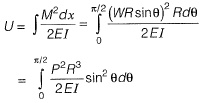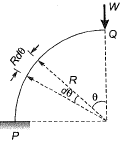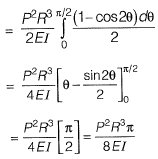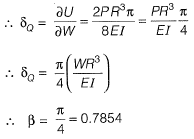Q.13 A simply supported beam is subjected to a uniformly distributed load of intensity w per unit length, on half of the span from one end. The length of the span and the flexural stiffness are denoted as I and EI respectively. The deflection at mid-span of the beam is [2012 : 2 Marks]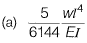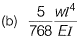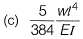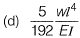Ans.
(B)
Solution:
Method-I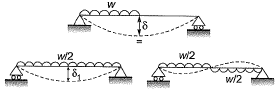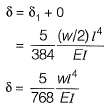Method-ll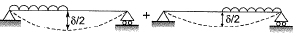Total deflection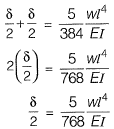Offer running on EduRev: Apply code STAYHOME200 to get INR 200 off on our premium plan EduRev Infinity!

69 docs

,

,

,

,

,

,

,

,

,

,

,

,

,

,

,

,

,

,

,

,

,

;